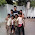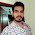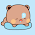Sunday, March 21, 2010

Bigint Library by Jan vai

Thanks to Jane Alam Jan vai for this, It will be a great help... :)

```/*
Author       :    Jan
Problem Name :    Big int for contest
Algorithm    :
Complexity   :
*/

#include <cstdio>
#include <string>
#include <algorithm>
using namespace std;

struct Bigint {
string a;
int sign;

Bigint() {}
Bigint( string b ) { (*this) = b; }
int size() { return a.size(); }
Bigint inverseSign() { sign *= -1; return (*this); }
Bigint normalize( int newSign ) {
sign = newSign;
for( int i = a.size() - 1; i > 0 && a[i] == '0'; i-- ) a.erase(a.begin() + i);
if( a.size() == 1 && a == '0' ) sign = 1;
return (*this);
}
void operator = ( string b ) {
a = b == '-' ? b.substr(1) : b;
reverse( a.begin(), a.end() );
this->normalize( b == '-' ? -1 : 1 );
}
bool operator < ( const Bigint &b ) const {
if( a.size() != b.a.size() ) return a.size() < b.a.size();
for( int i = a.size() - 1; i >= 0; i-- ) if( a[i] != b.a[i] ) return a[i] < b.a[i];
return false;
}
Bigint operator + ( Bigint b ) {
if( sign != b.sign ) return (*this) - b.inverseSign();
Bigint c;
for( int i = 0, carry = 0; i < (int)a.size() || i < (int)b.size() || carry; i++ ) {
carry += (i < (int)a.size() ? a[i] - 48 : 0) + (i < (int)b.a.size() ? b.a[i] - 48 : 0);
c.a += (carry % 10 + 48);
carry /= 10;
}
return c.normalize(sign);
}
Bigint operator - ( Bigint b ) {
if( sign != b.sign ) return (*this) + b.inverseSign();
if( (*this) < b ) return (b - (*this)).inverseSign();
Bigint c;
for( int i = 0, borrow = 0; i < (int)a.size(); i++ ) {
borrow = a[i] - borrow - (i < b.size() ? b.a[i] : 48);
c.a += borrow >= 0 ? borrow + 48 : borrow + 58;
borrow = borrow >= 0 ? 0 : 1;
}
return c.normalize(sign);
}
Bigint operator * ( Bigint b ) {
Bigint c("0");
for( int i = 0, k = a[i]; i < (int)a.size(); i++, k = a[i] ) {
while(k-- - 48) c = c + b;
b.a.insert(b.a.begin(), '0');
}
return c.normalize(sign * b.sign);
}
Bigint operator / ( Bigint b ) {
if( b.size() == 1 && b.a == '0' ) b.a /= ( b.a - 48 ) ;
Bigint c("0"), d;
for( int j = 0; j < (int)a.size(); j++ ) d.a += "0";
int dSign = sign * b.sign; b.sign = 1;
for( int i = a.size() - 1; i >= 0; i-- ) {
c.a.insert( c.a.begin(), '0');
c = c + a.substr( i, 1 );
while( !( c < b ) ) c = c - b, d.a[i]++;
}
return d.normalize(dSign);
}
Bigint operator % ( Bigint b ) {
if( b.size() == 1 && b.a == '0' ) b.a /= ( b.a - 48 ) ;
Bigint c("0");
int cSign = sign * b.sign; b.sign = 1;
for( int i = a.size() - 1; i >= 0; i-- ) {
c.a.insert( c.a.begin(), '0');
c = c + a.substr( i, 1 );
while( !( c < b ) ) c = c - b;
}
return c.normalize(cSign);
}
void print() {
if( sign == -1 ) putchar('-');
for( int i = a.size() - 1; i >= 0; i-- ) putchar(a[i]);
}
};

int main() {
Bigint a, b, c;
a = "511";
b = "10";

c = a + b;
c.print();
putchar('\n');

c = a - b;
c.print();
putchar('\n');

c = a * b;
c.print();
putchar('\n');

c = a / b;
c.print();
putchar('\n');

c = a % b;
c.print();
putchar('\n');

return 0;
}
```

Hope this helps my friends as well. Actually many of us are afraid of big integers, and also the above code may look hard, but this is actually what we do when performing these operations in real life... Use with caution, not a very first algorithm. Do not attempt to use *, /, % if numbers are 104 or more digits long.

1.Is it alright if I borrow this?

Truth be told, I'm afraid of bignums -- I don't like reinventing the wheel when a single mistake can skew the whole solution.

2.haha, Sure, I myself have borrowed it from some other one :)

3.I used this code in one of the problems and apparently the code was running too slow. When I ran the same code using Java BigInteger class, it was particularly fast, enough to get me an AC.
Since the only variation of parameter in my two codes was the BigInteger class, it seems that this code is slow :(.

4.@sidi, probably u missed the bottom of the post, I have written about the performance: "Use with caution, not a very first algorithm. Do not attempt to use *, /, % if numbers are 103 or more digits long."

5.brother, what would I have to write if I want to take input when the program is running?

6.Thanks Zobayer..for this great solution......now I can handle big Int in C++ as well...

1.These are just an outline regarding how to handle big ingegers in C/C++. But these are not efficient. But once you understand, you can write a more efficient version without much trouble. :)

7.Como posso usar na linguagem C